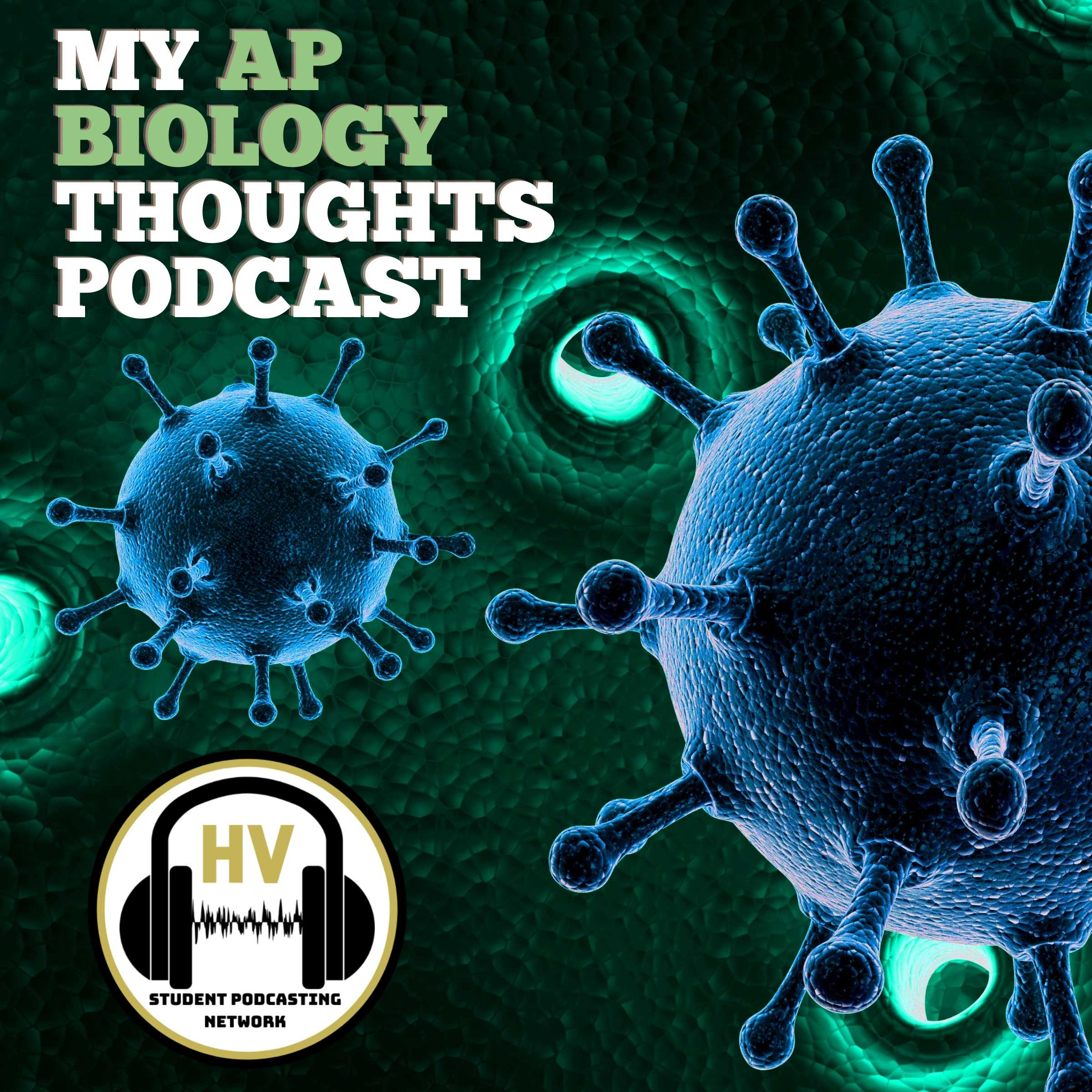Water Potential
Episode 6314th May 2021 • My AP Biology Thoughts • Hopewell Valley Student Publications Network
00:00:00 00:05:40

## Unit 2 Cell Structure and Function

Welcome to My AP Biology Thoughts podcast, my name is Pauline and I am your host for episode #63 called Unit 2 Cell Structure and Function: Water Potential. Today we will be discussing the basics of water potential in relation to cells.

## Segment 1: Introduction to Water Potential

•  There is a common misconception in differentiating osmosis and water potential. Osmosis is the movement of water through the semipermeable membrane. Water potential is the potential energy of water per unit area compared to pure water, so in simpler terms it allows us to figure out where water is going to flow. Water will always flow from high to low water potential. There are two equations to calculate it. First, water potential is equal to the solute potential plus the pressure potential. The solute potential is a factor of osmosis, while the pressure potential is a factor of pressure on a cell wall. The second equation comes into play to calculate the solute potential. The equation is -iCRT, where i is the ionization constant, C is the molar concentration, R is the pressure constant, and T is temperature in kelvin degrees. The units are bars.

Segment 2: More About Water Potential

•  Most of the water potential problems you will be presented with will be straightforward algebra to plug into the solute potential equation to then determine the net flow of the water. For example, it is given that a cell’s pressure potential is 3 bars and the solute potential is -4.5 bars. The cell is placed in an open beaker of sugar water with a solute potential of -4 bars and you are asked to determine the net flow of the water. You would find the cell’s water potential by adding 3 and -4.5 bars to get -1.5 bars, which is greater than the water potential of the sugar water, so the water will flow out of the cell. A key takeaway from this example is that pressure potential in an open beaker will always be zero. Another example could give you the same information, but the cell was placed in an NaCl solution instead, which therefore changes the ionization constant to 2 because there are now 2 ions in the solution. Lastly, the most complex example would involve cubes of vegetables like potatoes or zucchini to be placed in sucrose solutions with different concentrations. The percent change in mass of each cube would be calculated after the experiment held at a constant temperature. It will ask you to calculate the water potential of the cubes. The best way to come around these problems is to graph the sucrose concentrations versus the percent change of mass. This will result in a linear relationship, where the x-intercept represents the cell in an isotonic state when equal amounts of water are leaving and entering. So you would then plug in the value of the concentration at the x-intercept into the iCRT equation and go from there.

## Segment 3: Connection to the Course

• Water potential is a concept to have mastered because it explains the most basic observations. It explains why humans cannot drink salt water because since seawater has a lower water potential than our cells, drinking it will only cause water to flow out of our cells and into the seawater. This ultimately will kill our cells because they will shrivel up and shrink, which reduces your chances of survival. On the other hand, it also explains why red blood cells cannot be found in distilled water. The water would go into the more hypertonic red blood cell causing it to swell and then burst. Overall, water potential explains the results of isotonic, hypertonic, and hypotonic solutions all due to osmosis.

Thank you for listening to this episode of My AP Biology Thoughts. For more student-ran podcasts and digital content, make sure that you visit www.hvspn.com. See you next time!

## Music Credits:

• “Ice Flow” Kevin MacLeod (incompetech.com)